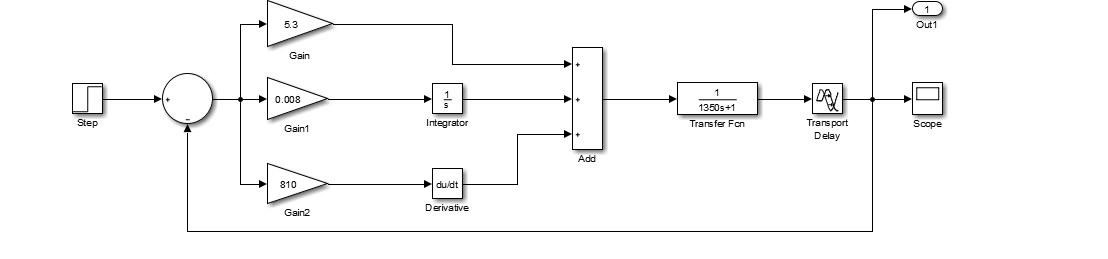2个回答

plotyy（T,I,T,V）就行 这条语句是很简单的左边的纵轴就是电流，右边的纵轴就是Vgt0808 你在说什么，根本就是答非所问。
8 个月之前 回复matlab simulink 可以有两个simulation type吗？ 我的一个元件需要选择phasor， 另一个需要选择discrete。这样该如何处理？

1、按照书上给出的步骤执行，但是simulink仿真出现问题，可能是传递函数转 化成模型时出错，不能正确执行。 2、代码如下： clear a11 clc % % % % % % % % % %电动机功率估算 m=1850; f=0.005;cd=0.49;A=3.8; vmax=60;i=0.1;vi=15; pv=(m*9.8*f/3600*vmax+cd*A/76140*vmax^3)/0.92; po=(m*9.8*f/3600*vi+cd*A/76140*vi^3+m*9.8*sin(atan(i))*vi/3600)/0.92; if pv>po p=pv; else p=po; end % % % % % % % % % %电动机驱动系统参数设计计算 R=0.025; %电枢电阻 L=0.018; %电枢电感 u=120; %额定电压 I=85; %额定电流 n=1500; %额定转速 ce=(u-R*I)/n; %电动机电动势系数0.04343 GD2=3.53; %飞轮转矩 cm=30/pi*ce; tm=GD2*R/375/cm/ce; %电动机时间常数 t1=L/R; %电枢回路电磁时间常数 ks=7.9; %触发整流装置放大系数 ts=0.0000667; %触发整流装置延迟系数，开关频率15KHZ alpha=10/1500; %转速反馈系数 beta=0.0908; %电流反馈系数 toi=0.002; %电流环滤波时间常数 t1=0.72; taoi=t1;%0.05; TI=ts+toi; KI=1/(2*TI); %电流开环增量 ki=KI*taoi*R/beta/ks; %电流调节器比例系数5.21 ton=0.2; Tn=2*TI+ton; taon=0.1207; h=5; KN=(h+1)/(2*h^2*Tn^2); %转速开环增益 kn=(h+1)*beta*ce*tm/(2*h*alpha*R*Tn); %转速调节器比例系数?? taodn=Tn*((4*h+2)/(h+1)); %?? [a,b,c,d]=linmod('diandong_che_double_loop'); sy=ss(a,b,c,d); %将系统的simulink结构图转换为状态空间模型 g=tf(sy); step(g,1); den=g.den{1}; %从传递函数中提取分母 num=g.num{1}; %从传递函数中提取分子 finals=polyval(num,0)/polyval(den,0); %求系统的稳态值 [y,t]=step(g); [ym,jmax]=max(y); Mp=100*(ym-finals)/finals; %求系统响应最大超调量 fprintf('电动车辆需求功率为= %3.2f KW\n',p) fprintf('系统最大超调量Mp= %3.3f% % \n',Mp) tp=t(jmax); fprintf('系统峰值时间tp=%3.3f s\n',tp) lt=length(t); while(y(lt)>0.98*finals)&&(y(lt)<1.02*finals) lt=lt-1; end tr=t(lt); fprintf('系统调整时间（2% % ）tr=%3.3f s\n',tr )

Java校招入职华为，半年后我跑路了

Java基础知识面试题（2020最新版）

@程序员：GitHub这个项目快薅羊毛

C++(继承):19---虚基类与虚继承（virtual）

loonggg读完需要3分钟速读仅需 1 分钟大家好，我是你们的校长。我之前讲过，这年头，只要肯动脑，肯行动，程序员凭借自己的技术，赚钱的方式还是有很多种的。仅仅靠在公司出卖自己的劳动时...

win10暴力查看wifi密码

MySQL数据库面试题（2020最新版）

!大部分程序员只会写3年代码

2020阿里全球数学大赛：3万名高手、4道题、2天2夜未交卷